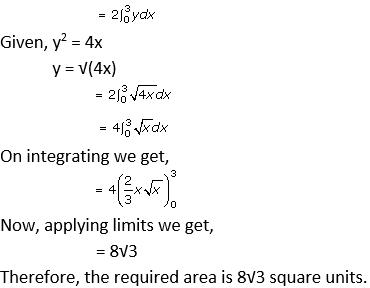# RD Sharma Solutions Class 12 Area Bounded Regions Exercise 21.1

RD Sharma Solutions for Class 12 Maths Exercise 21.1 Chapter 21 Areas of Bounded Regions are given here. The foremost objective is to help students understand and crack these questions. Students obtain more knowledge by referring to RD Sharma Solutions for Class 12 Maths Chapter 21. Download pdf of Class 12 Chapter 21 in their respective links.

## Download PDF of RD Sharma Solutions for Class 12 Maths Chapter 21 Exercise 1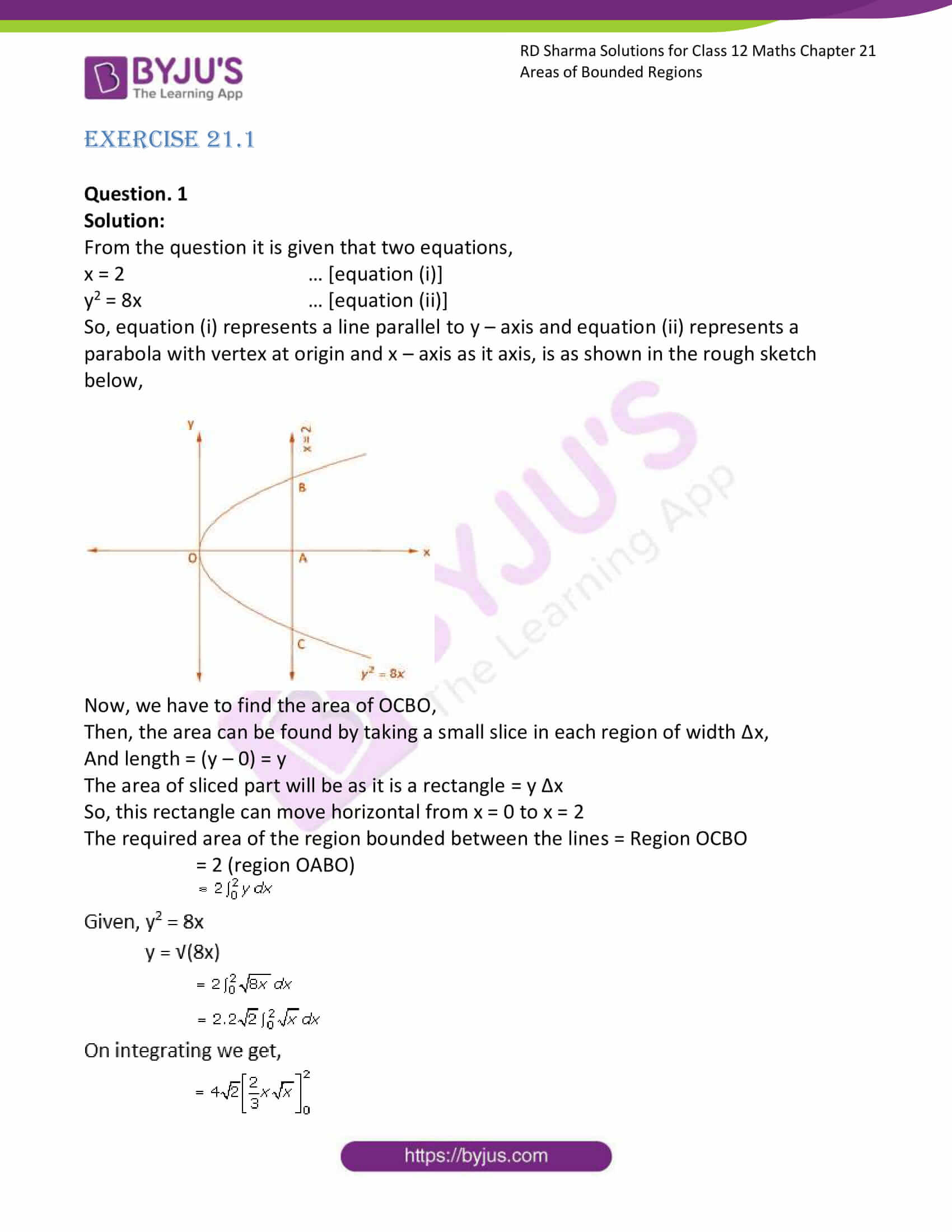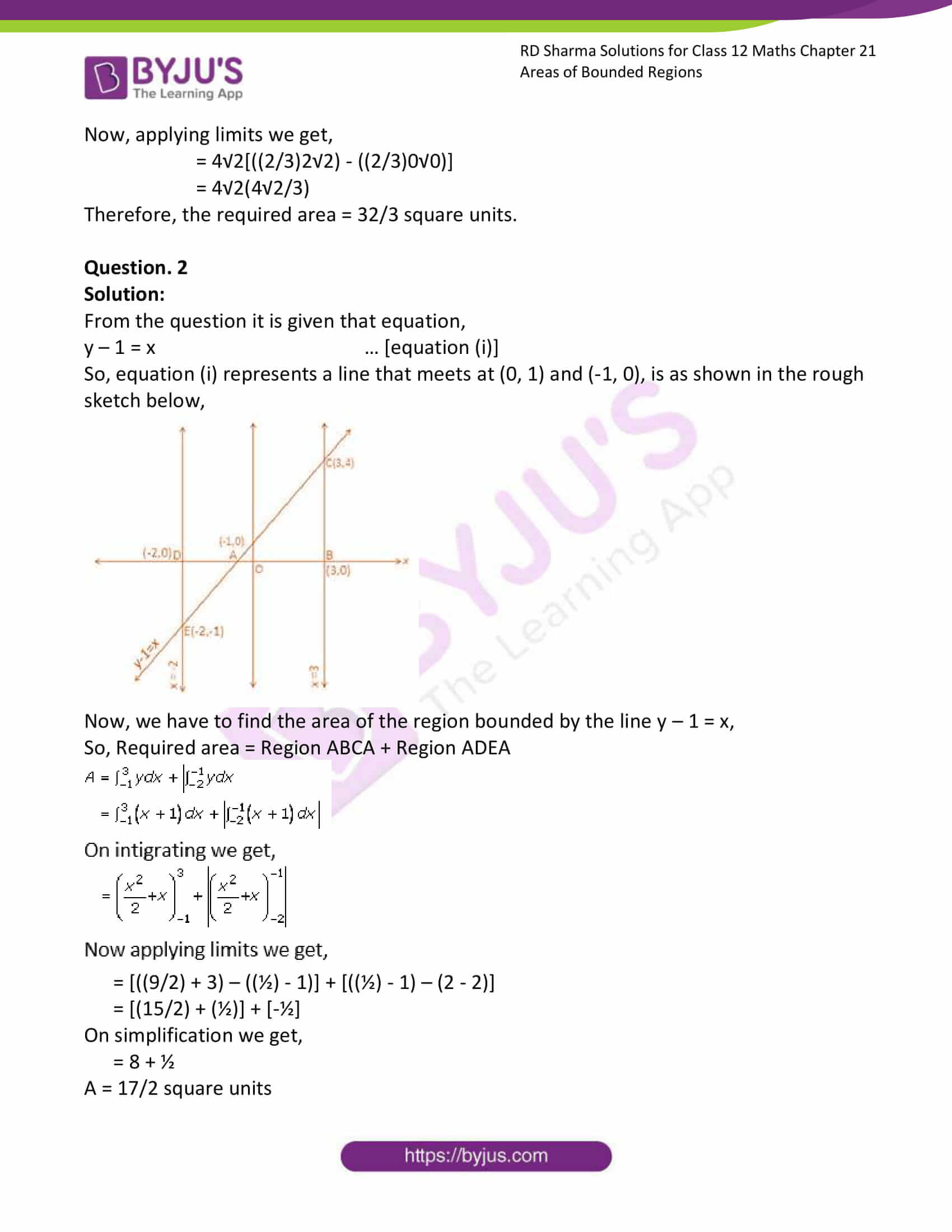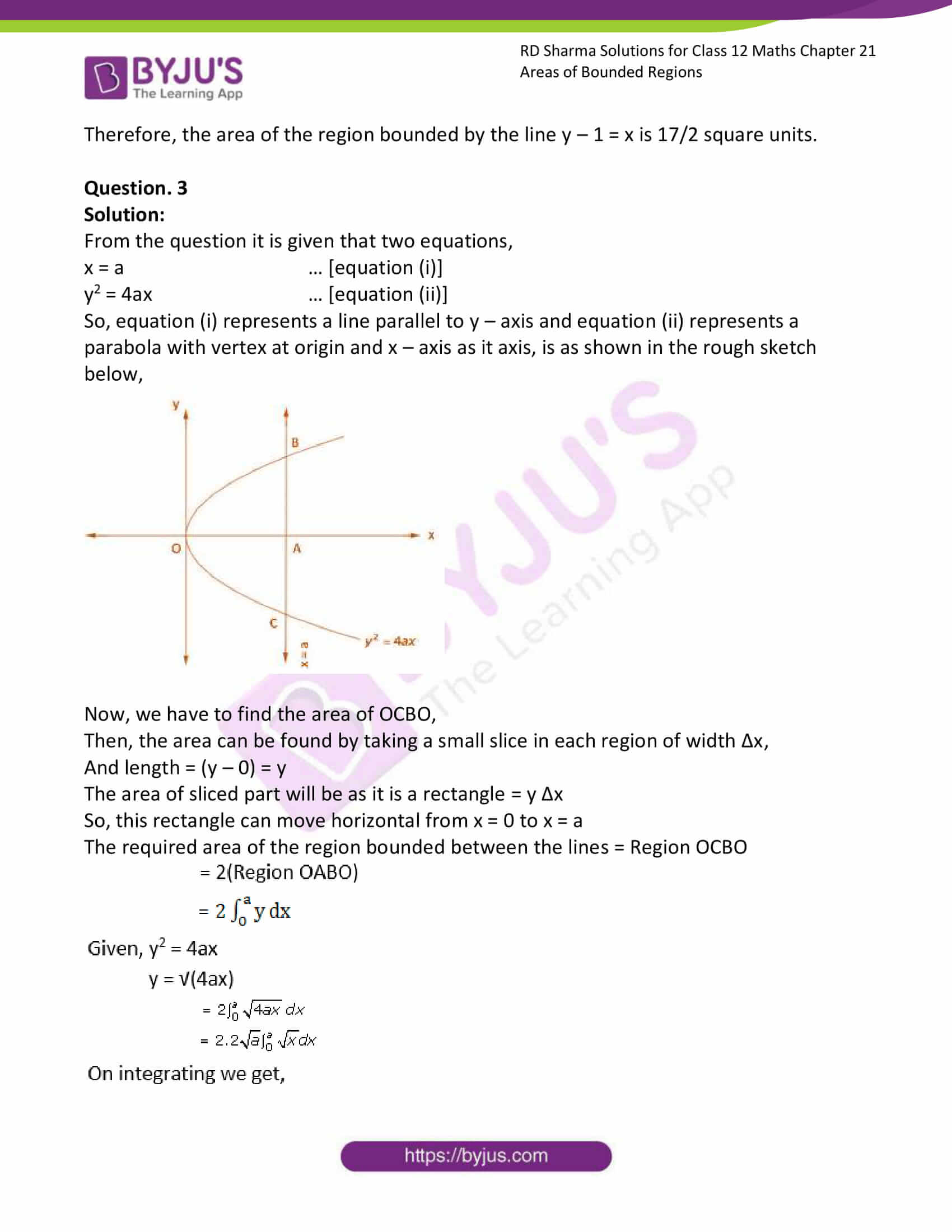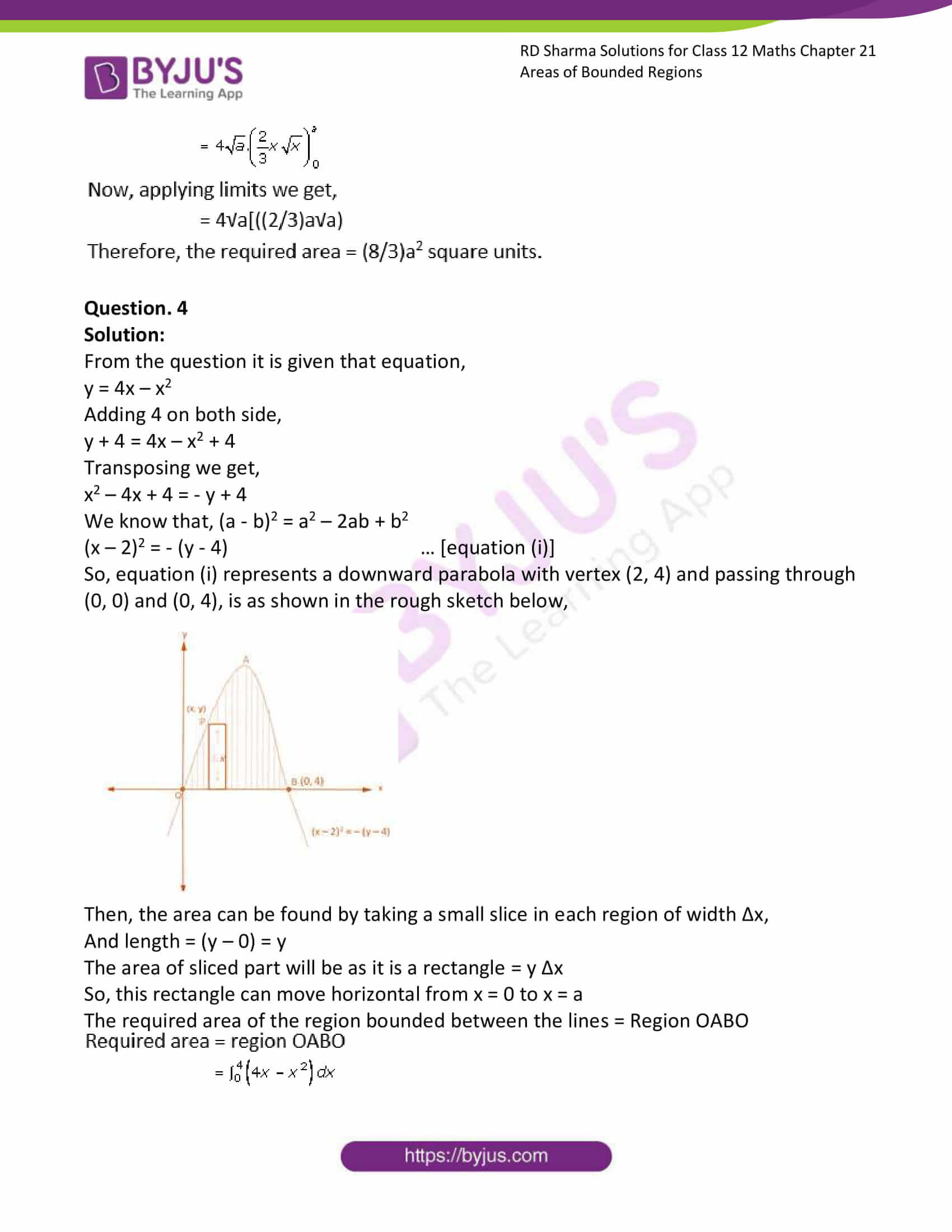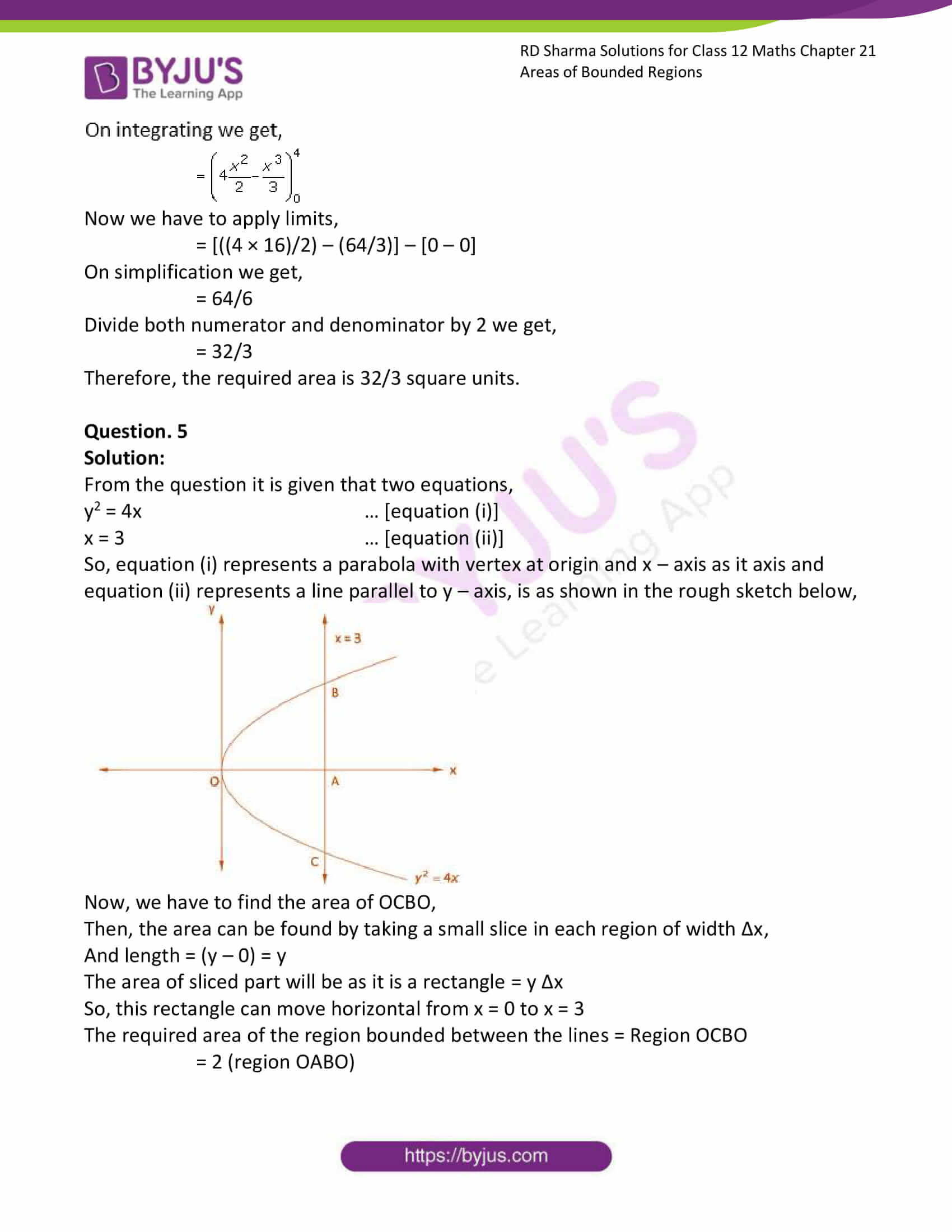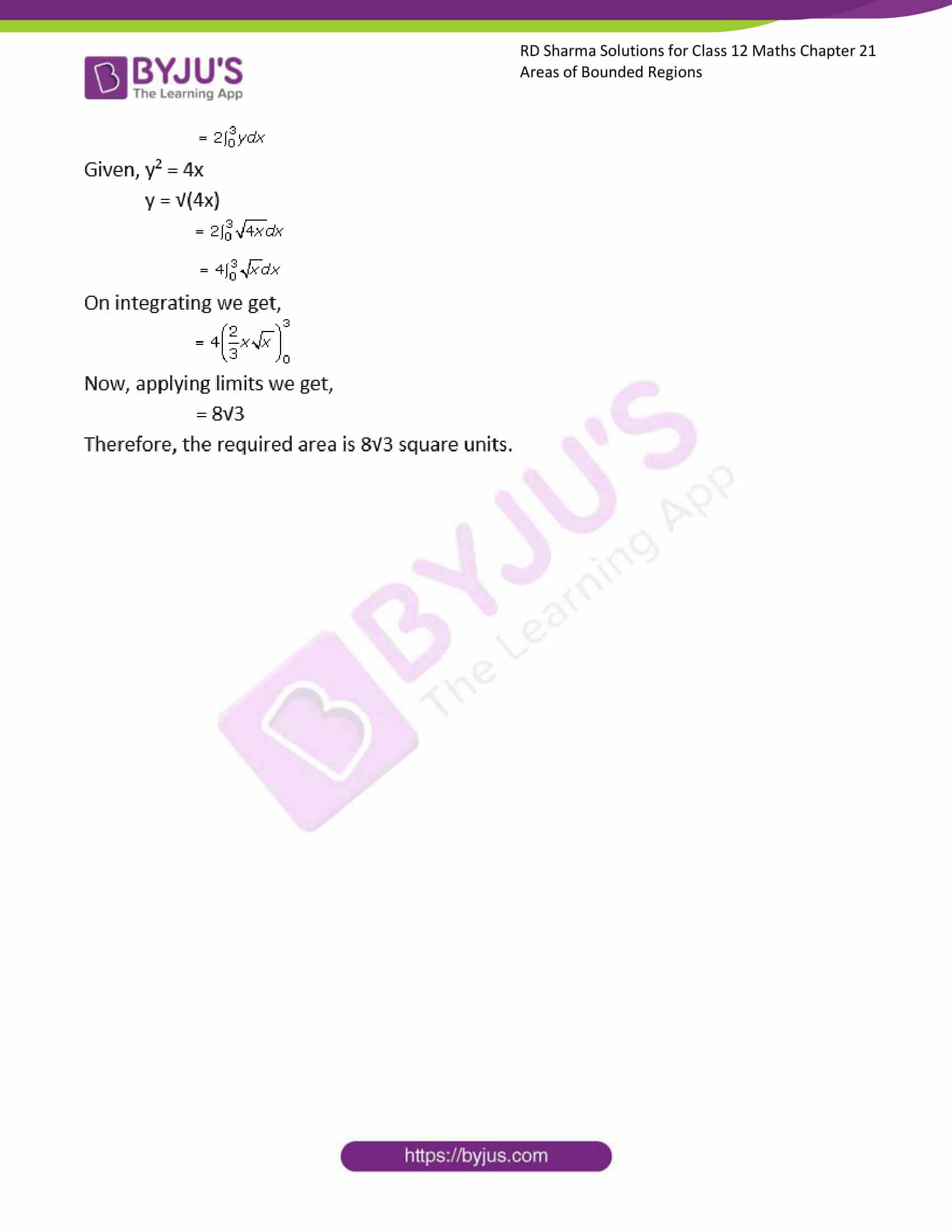### Access RD Sharma Solutions for Class 12 Maths Chapter 21 Exercise 1

EXERCISE 21.1

Question. 1

Solution:

From the question it is given that two equations,

x = 2 … [equation (i)]

y2 = 8x … [equation (ii)]

So, equation (i) represents a line parallel to y – axis and equation (ii) represents a parabola with vertex at origin and x – axis as it axis, is as shown in the rough sketch below,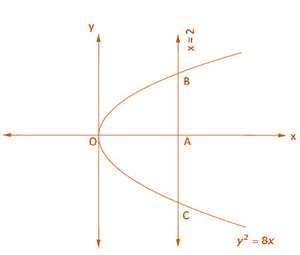Now, we have to find the area of OCBO,

Then, the area can be found by taking a small slice in each region of width Δx,

And length = (y – 0) = y

The area of sliced part will be as it is a rectangle = y Δx

So, this rectangle can move horizontal from x = 0 to x = 2

The required area of the region bounded between the lines = Region OCBO

= 2 (region OABO)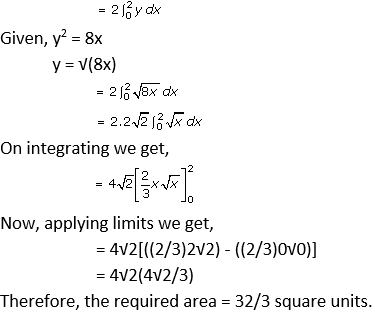Now, applying limits we get,

= 4√2[((2/3)2√2) – ((2/3)0√0)]

= 4√2(4√2/3)

Therefore, the required area = 32/3 square units.

Question. 2

Solution:

From the question it is given that equation,

y – 1 = x … [equation (i)]

So, equation (i) represents a line that meets at (0, 1) and (-1, 0), is as shown in the rough sketch below,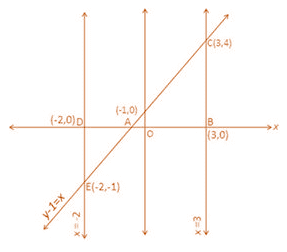Now, we have to find the area of the region bounded by the line y – 1 = x,

So, Required area = Region ABCA + Region ADEA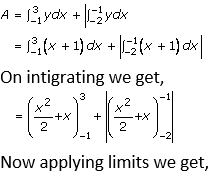= [((9/2) + 3) – ((½) – 1)] + [((½) – 1) – (2 – 2)]

= [(15/2) + (½)] + [-½]

On simplification we get,

= 8 + ½

A = 17/2 square units

Therefore, the area of the region bounded by the line y – 1 = x is 17/2 square units.

Question. 3

Solution:

From the question it is given that two equations,

x = a … [equation (i)]

y2 = 4ax … [equation (ii)]

So, equation (i) represents a line parallel to y – axis and equation (ii) represents a parabola with vertex at origin and x – axis as it axis, is as shown in the rough sketch below,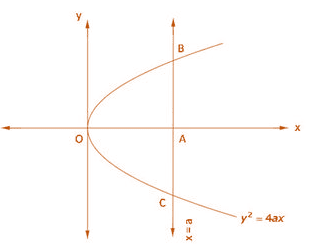Now, we have to find the area of OCBO,

Then, the area can be found by taking a small slice in each region of width Δx,

And length = (y – 0) = y

The area of sliced part will be as it is a rectangle = y Δx

So, this rectangle can move horizontal from x = 0 to x = a

The required area of the region bounded between the lines = Region OCBO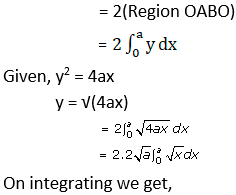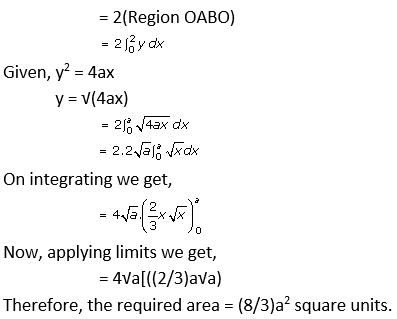Question. 4

Solution:

From the question it is given that equation,

y = 4x – x2

y + 4 = 4x – x2 + 4

Transposing we get,

x2 – 4x + 4 = – y + 4

We know that, (a – b)2 = a2 – 2ab + b2

(x – 2)2 = – (y – 4) … [equation (i)]

So, equation (i) represents a downward parabola with vertex (2, 4) and passing through (0, 0) and (0, 4), is as shown in the rough sketch below,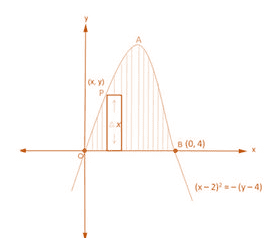Then, the area can be found by taking a small slice in each region of width Δx,

And length = (y – 0) = y

The area of sliced part will be as it is a rectangle = y Δx

So, this rectangle can move horizontal from x = 0 to x = a

The required area of the region bounded between the lines = Region OABO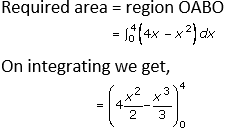Now we have to apply limits,

= [((4 × 16)/2) – (64/3)] – [0 – 0]

On simplification we get,

= 64/6

Divide both numerator and denominator by 2 we get,

= 32/3

Therefore, the required area is 32/3 square units.

Question. 5

Solution:

From the question it is given that two equations,

y2 = 4x … [equation (i)]

x = 3 … [equation (ii)]

So, equation (i) represents a parabola with vertex at origin and x – axis as it axis and equation (ii) represents a line parallel to y – axis, is as shown in the rough sketch below,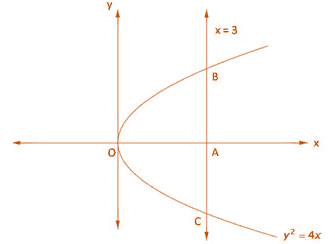Now, we have to find the area of OCBO,

Then, the area can be found by taking a small slice in each region of width Δx,

And length = (y – 0) = y

The area of sliced part will be as it is a rectangle = y Δx

So, this rectangle can move horizontal from x = 0 to x = 3

The required area of the region bounded between the lines = Region OCBO

= 2 (region OABO)